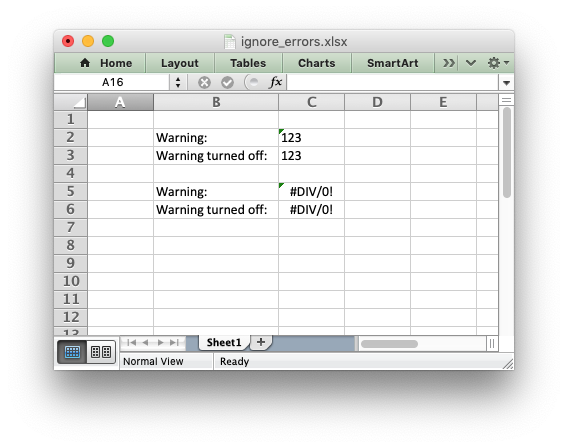# Example: Ignoring Worksheet errors and warnings#

An example of ignoring Excel worksheet errors/warnings using the worksheet `ignore_errors()` method.```##############################################################################
#
# An example of turning off worksheet cells errors/warnings using the
# XlsxWriter Python module.
#
# Copyright 2013-2023, John McNamara, jmcnamara@cpan.org
#
import xlsxwriter

workbook = xlsxwriter.Workbook("ignore_errors.xlsx")

# Write strings that looks like numbers. This will cause an Excel warning.
worksheet.write_string("C2", "123")
worksheet.write_string("C3", "123")

# Write a divide by zero formula. This will also cause an Excel warning.
worksheet.write_formula("C5", "=1/0")
worksheet.write_formula("C6", "=1/0")

# Turn off some of the warnings:
worksheet.ignore_errors({"number_stored_as_text": "C3", "eval_error": "C6"})

# Write some descriptions for the cells and make the column wider for clarity.
worksheet.set_column("B:B", 16, None)
worksheet.write("B2", "Warning:")
worksheet.write("B3", "Warning turned off:")
worksheet.write("B5", "Warning:")
worksheet.write("B6", "Warning turned off:")

workbook.close()
```